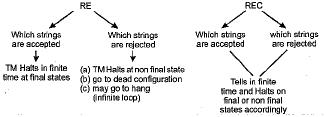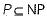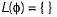Courses

# Turing Machine: RE, REC And Undecidability (Basic Level) - 1

## 10 Questions MCQ Test Question Bank for GATE Computer Science Engineering | Turing Machine: RE, REC And Undecidability (Basic Level) - 1

Description
This mock test of Turing Machine: RE, REC And Undecidability (Basic Level) - 1 for Computer Science Engineering (CSE) helps you for every Computer Science Engineering (CSE) entrance exam. This contains 10 Multiple Choice Questions for Computer Science Engineering (CSE) Turing Machine: RE, REC And Undecidability (Basic Level) - 1 (mcq) to study with solutions a complete question bank. The solved questions answers in this Turing Machine: RE, REC And Undecidability (Basic Level) - 1 quiz give you a good mix of easy questions and tough questions. Computer Science Engineering (CSE) students definitely take this Turing Machine: RE, REC And Undecidability (Basic Level) - 1 exercise for a better result in the exam. You can find other Turing Machine: RE, REC And Undecidability (Basic Level) - 1 extra questions, long questions & short questions for Computer Science Engineering (CSE) on EduRev as well by searching above.
QUESTION: 1

### Which of the following functions are computable with Turning Machine?

Solution:

All the above functions are total recursive functions. The total recursive functions are like recursive language. Turing machine halts on each and every input of recursive language. All of the above functions are turing computable.

QUESTION: 2

### A countable union of countable sets is not

Solution:

A countable union of countable set is countable. Countably infinite and denumerable are alternate names of countable sets.

QUESTION: 3

### ∑* over {0,1} and 2∑* are respectively,

Solution:

Since ∑* is all the combinations of 0 and 1 and yet it is enumerable and belongs to the category of countably infinite. But 2∑* is the power set of ∑* which is infinite. By diagonalisation theorem, power set of any countably infinite set is always uncountable, hence 2∑*​ belongs to uncountably infinite set.

QUESTION: 4

Which of the following is undecidable?

Solution:

According to the decision properties of the CFL and Regular languages, Equivalence of regular languages and emptiness of regular languages are decidable. Also the finiteness of CFL is decidable. For each of above property an algorithm exist. But no algorithm exist for checking the equivalence of the two context-free language.

QUESTION: 5

The statement "ATM can’t solve halting problem’’ is

Solution:

ATM cannot solves the halting problem because it cannot find in finite time that for the given word the machine will halt or not.

QUESTION: 6

A PDA behaves like a TM when the number of auxiliary memory it has, is

Solution:

Auxiliary memory = memory used by the machine for calculations or swappings.
PDA with 2 or more stacks is equivalent to TM.

QUESTION: 7

Halting problem/language is

Solution:

Halting problem belongs to the TM. The language in which halting problem belongs is recursively enumerable i.e. RE. In this TM can’t tell in finite time the word is rejected or not. Although it can tell in finite time that the given word is accepted or not. Although REC can tell in finite time and comes into halt about both i.e.QUESTION: 8

The statementis

Solution:

The statementis always true.

QUESTION: 9

If e1 and e2 are the RE denoting the languages Land L2 respectively, then which of the following is wrong?

Solution:

Choice (c) is wrong since “φ” is a regular. In fact*Multiple options can be correct
QUESTION: 10

Which of the following is in P?
PATH, HAMPATH, SAT, 3SAT

Solution:

SAT and PATH is in P while 3SAT and HAMPATH are NPC.